# 一文讀懂！異常檢測全攻略！從統計方法到機器學習 ⛵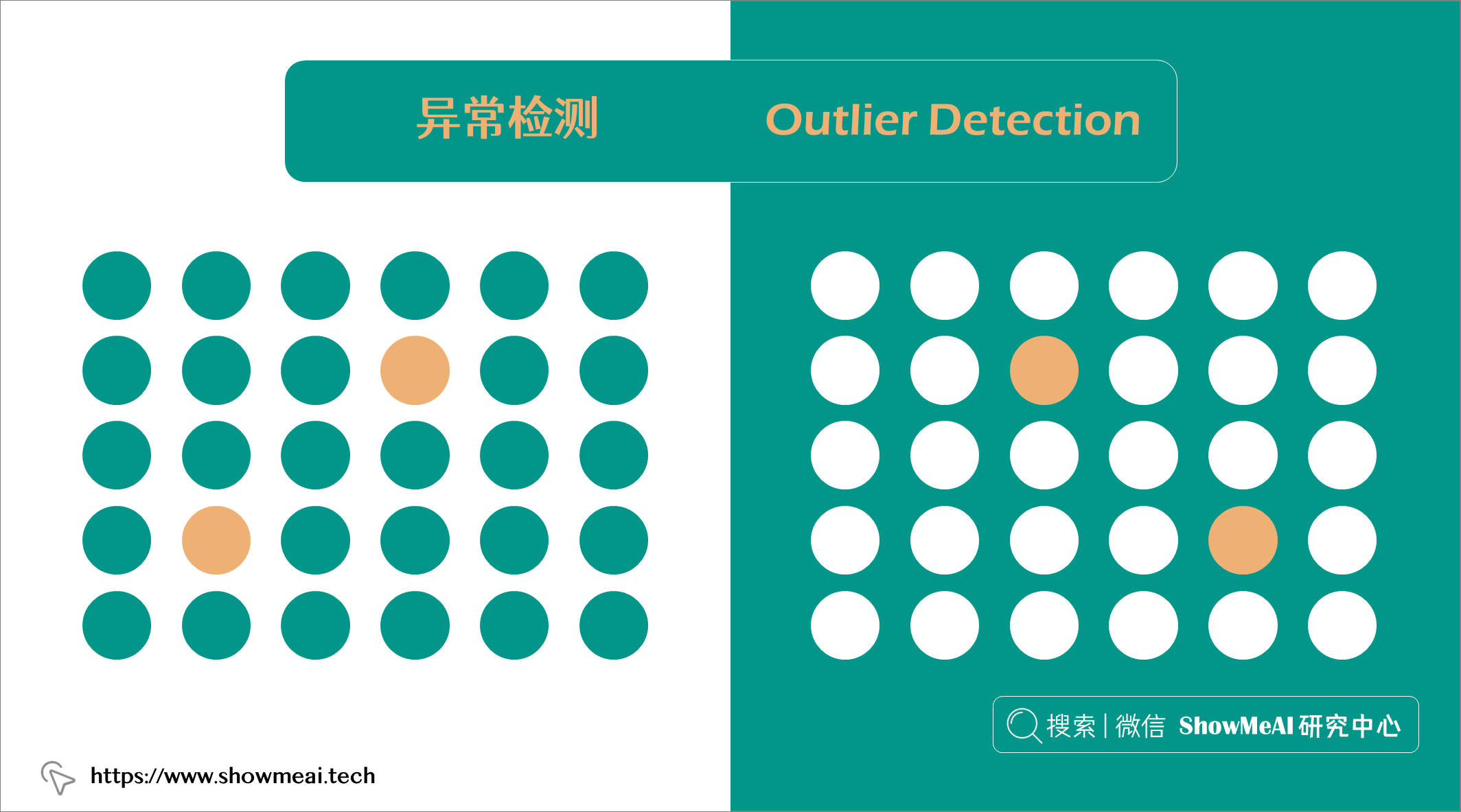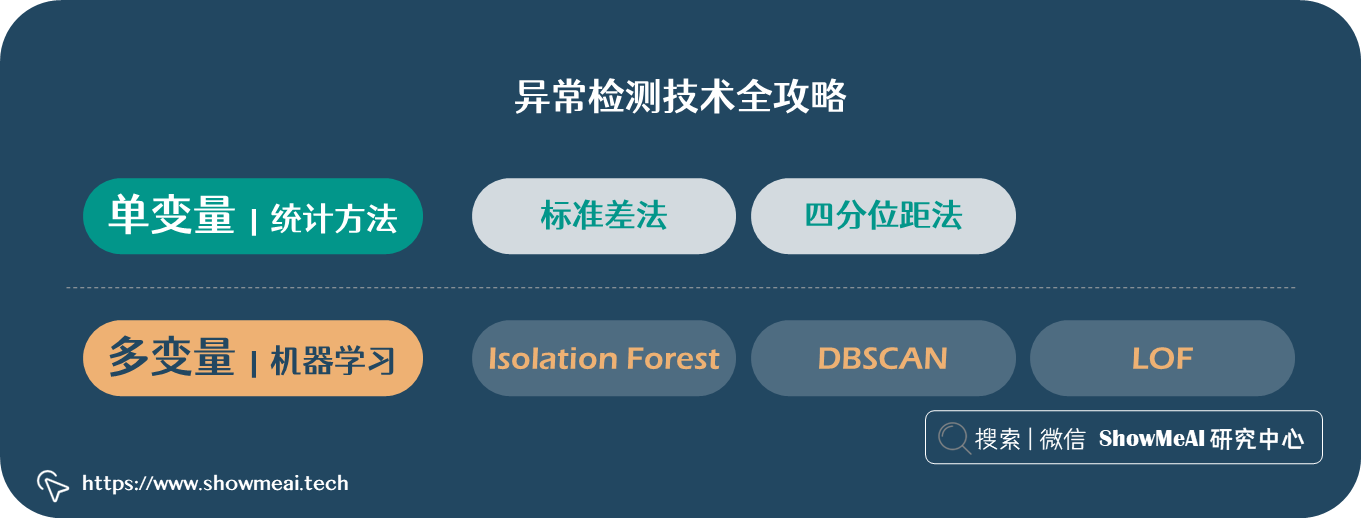# 💡 資料&場景方法概述

## 💦 資料

🏆 實戰資料集下載（百度網盤）：公眾號『ShowMeAI研究中心』回覆『實戰』，或者點選 這裡 獲取本文 異常檢測實戰全景圖：從統計方法到機器學習glass資料集

ShowMeAI官方GitHubhttps://github.com/ShowMeAI-Hub

```python import pandas as pd glass = pd.read_csv('glass.csv')```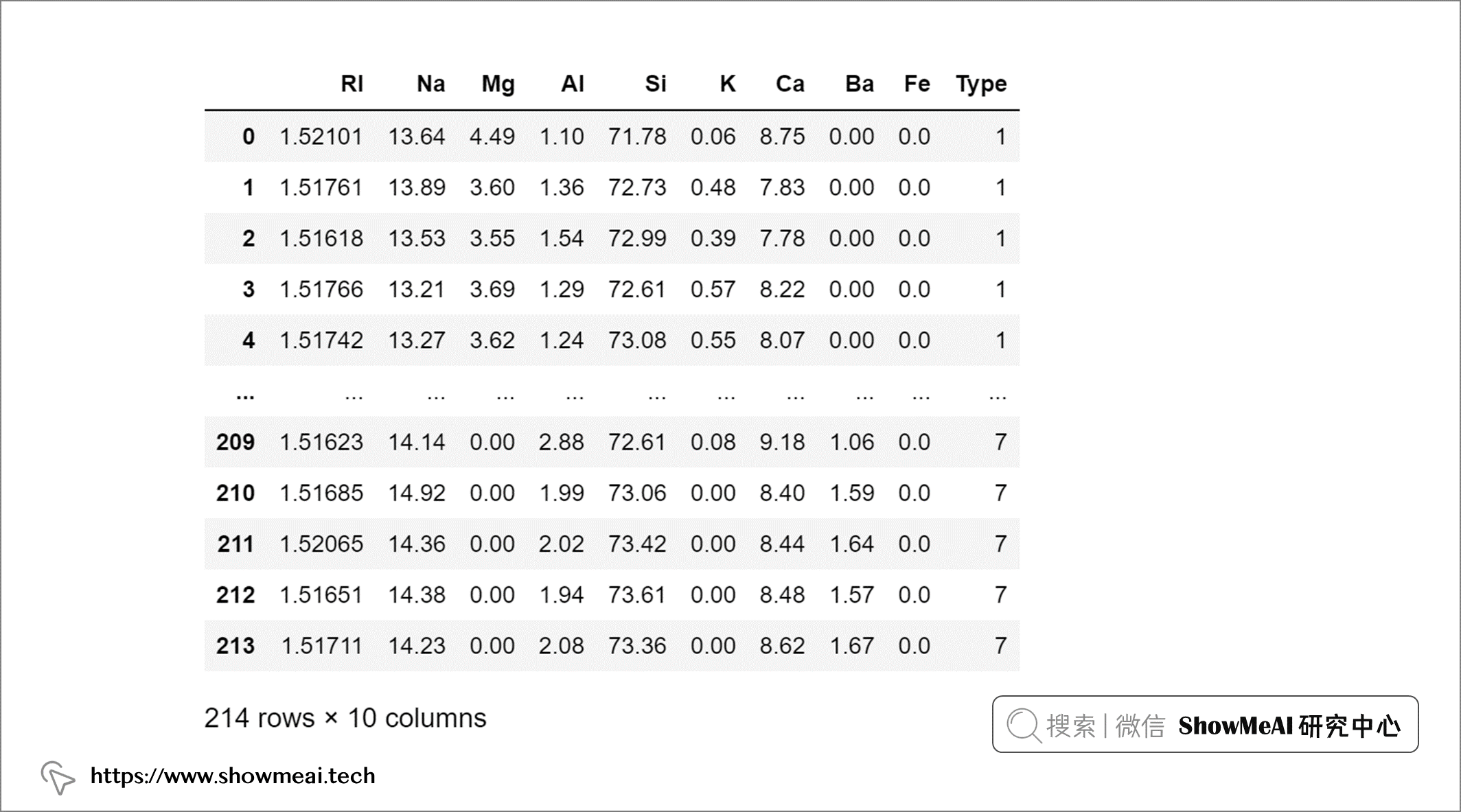## 💦 單變數和多變數異常值```python import seaborn as sns sns.pairplot(glass, diag_kws={'color':'red'})```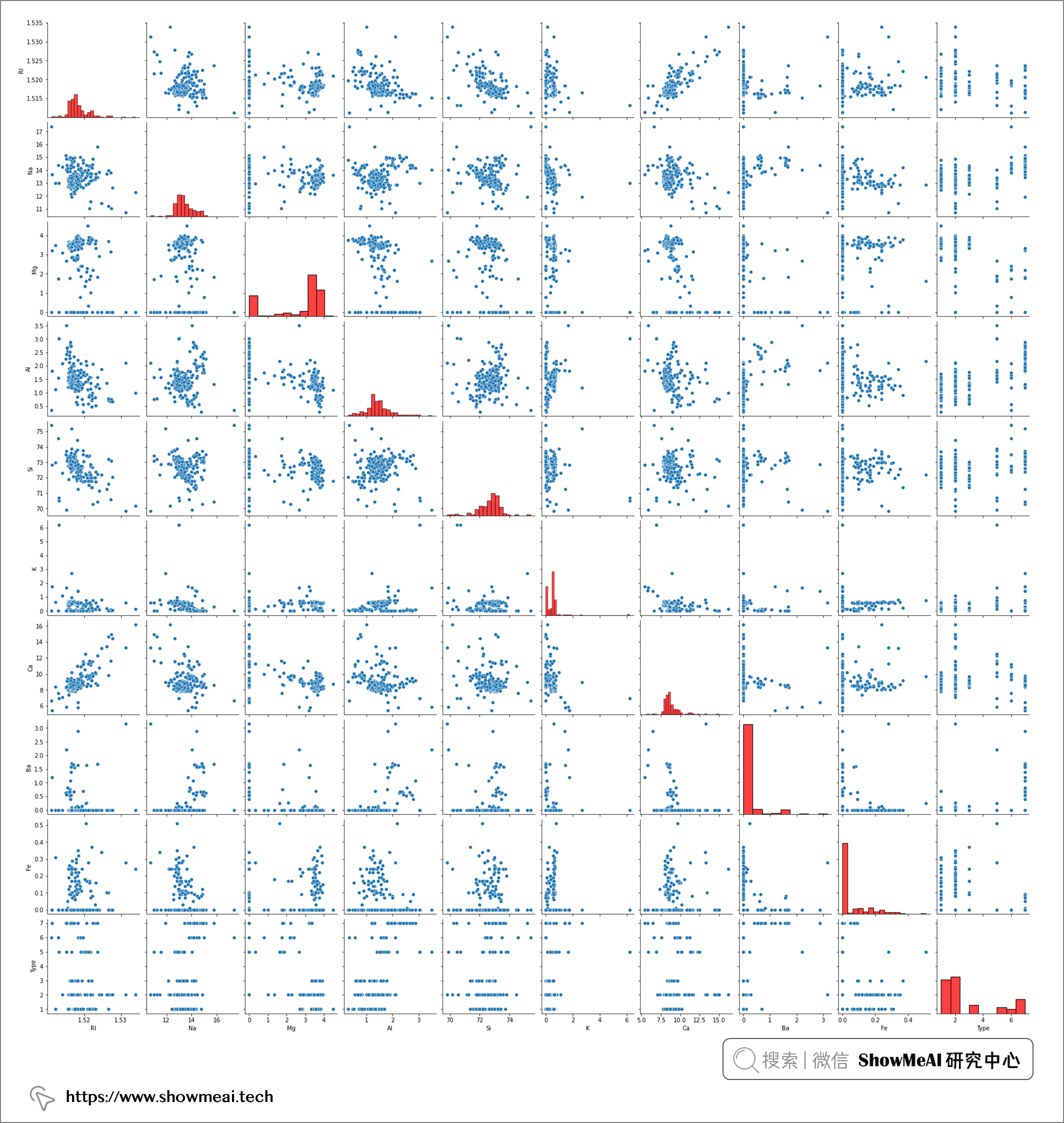pairplot 的結果包含兩兩資料的關聯分析和每個變數的分佈結果，其中對角線為單變數的分佈視覺化，我們發現並非所有屬性欄位都具有遵循正態分佈。事實上，大多數屬性都偏向較低值（即 Ba、Fe*）或較高值（即 Mg）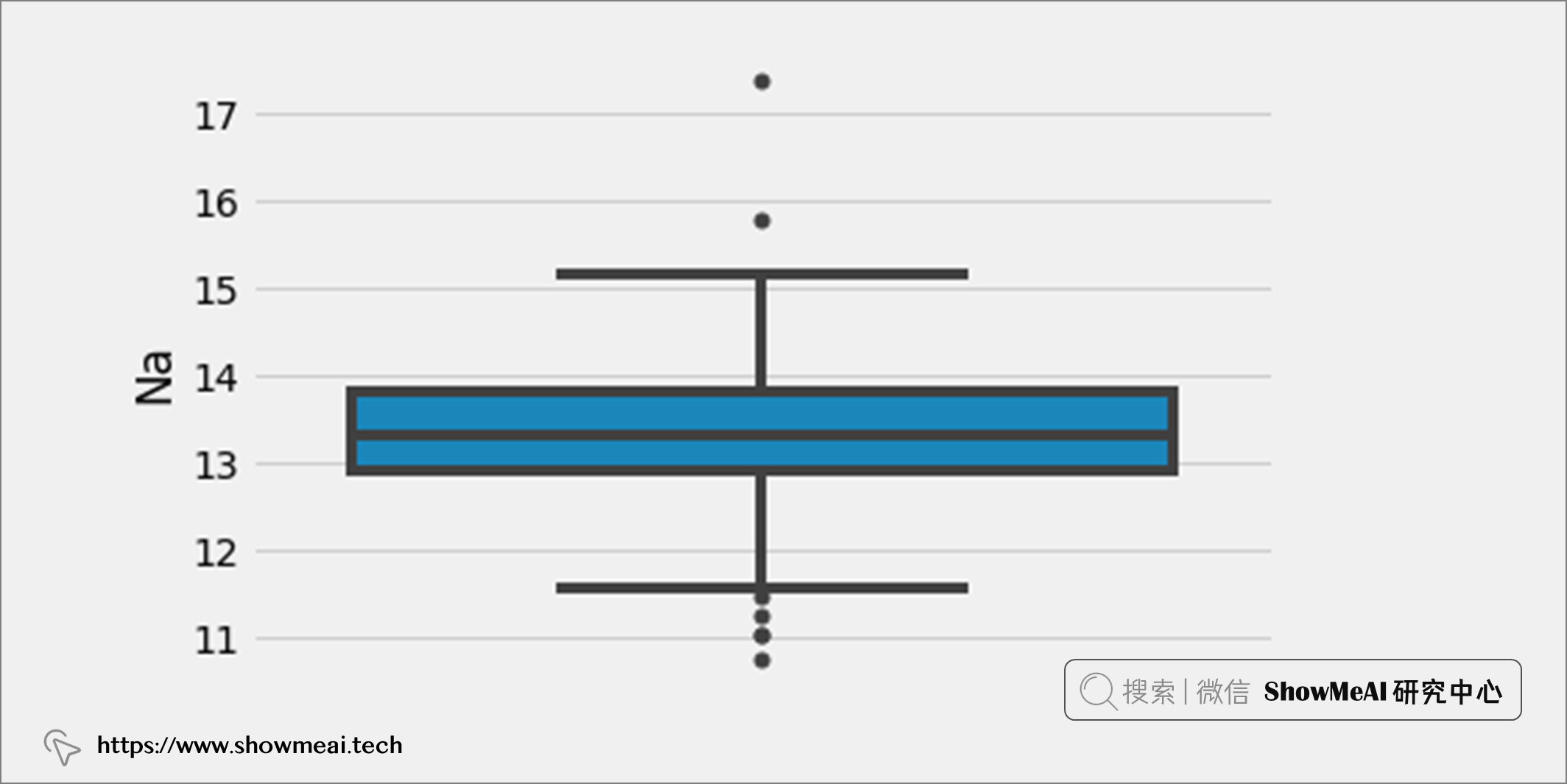```python from sklearn.decomposition import PCA import plotly.express as px

# Dimensionality reduction to 3 dimensions

pca = PCA(n_components=3) glass_pca = pca.fit_transform(glass.iloc[:, :-1])

# 3D scatterplot

fig = px.scatter_3d(x=glass_pca[:, 0], y=glass_pca[:, 1], z=glass_pca[:, 2], color=glass.iloc[:, -1]) fig.show() ```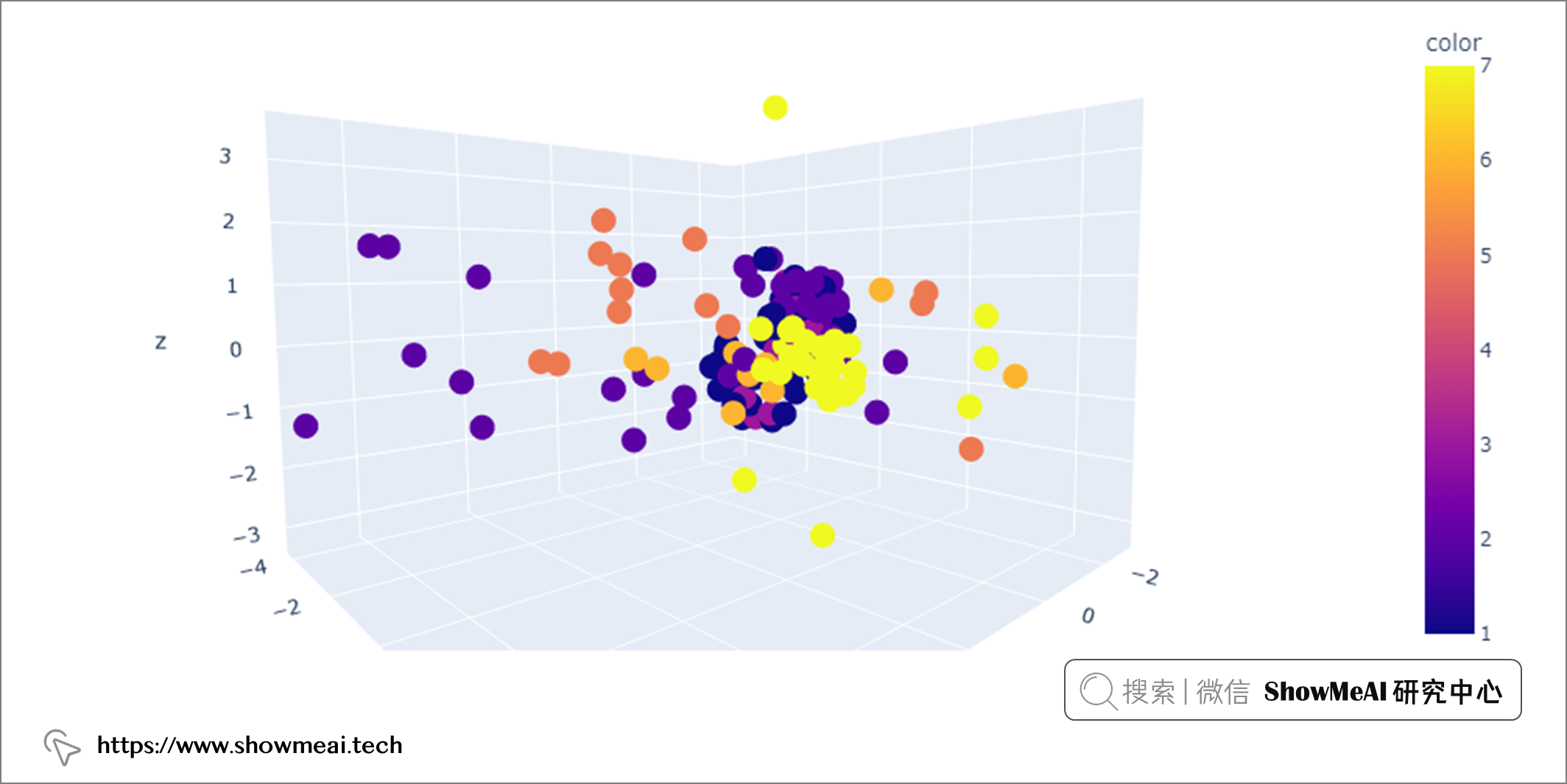# 💡 單變數異常值檢測

## 💦 標準差法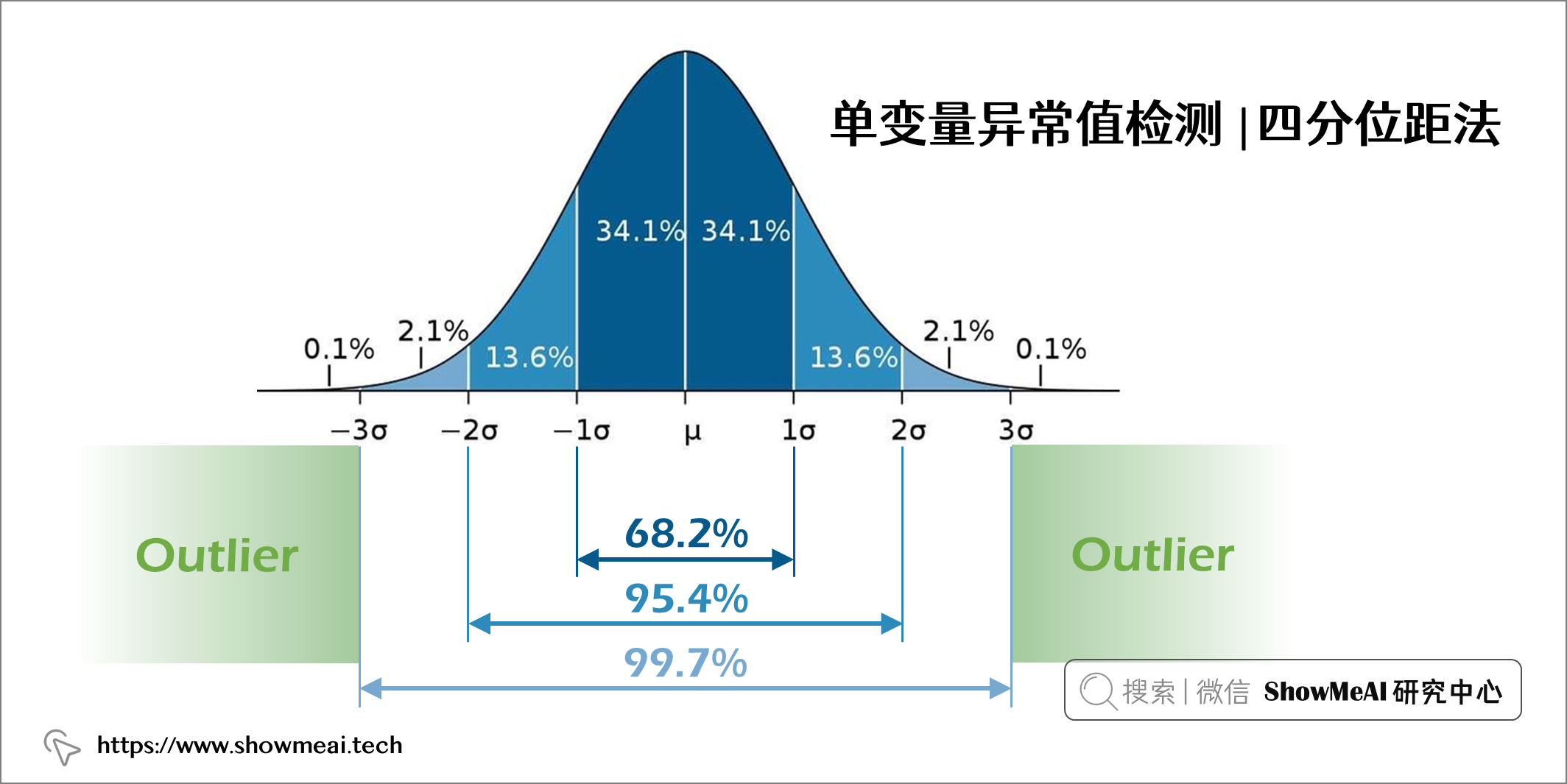```python

# Find mean, standard deviation and cut off value

mean = glass["Na"].mean() std = glass["Na"].std() cutoff = 3 * std

# Define lower and upper boundaries

lower, upper = mean-cutoff, mean+cutoff

# Define new dataset by masking upper and lower boundaries

new_glass = glass[(glass["Na"] > lower) & (glass["Na"] < upper)] ```

```Shape of original dataset: (213, 9) Shape of dataset after removing outliers in Na column: (211, 9)```

## 💦 四分位距法

• 四分位數 1 (Q1) 表示第 25 個百分位數
• 四分位數 2 (Q2) 表示第 50 個百分位數
• 四分位數 3 (Q3) 表示第 75 個百分位數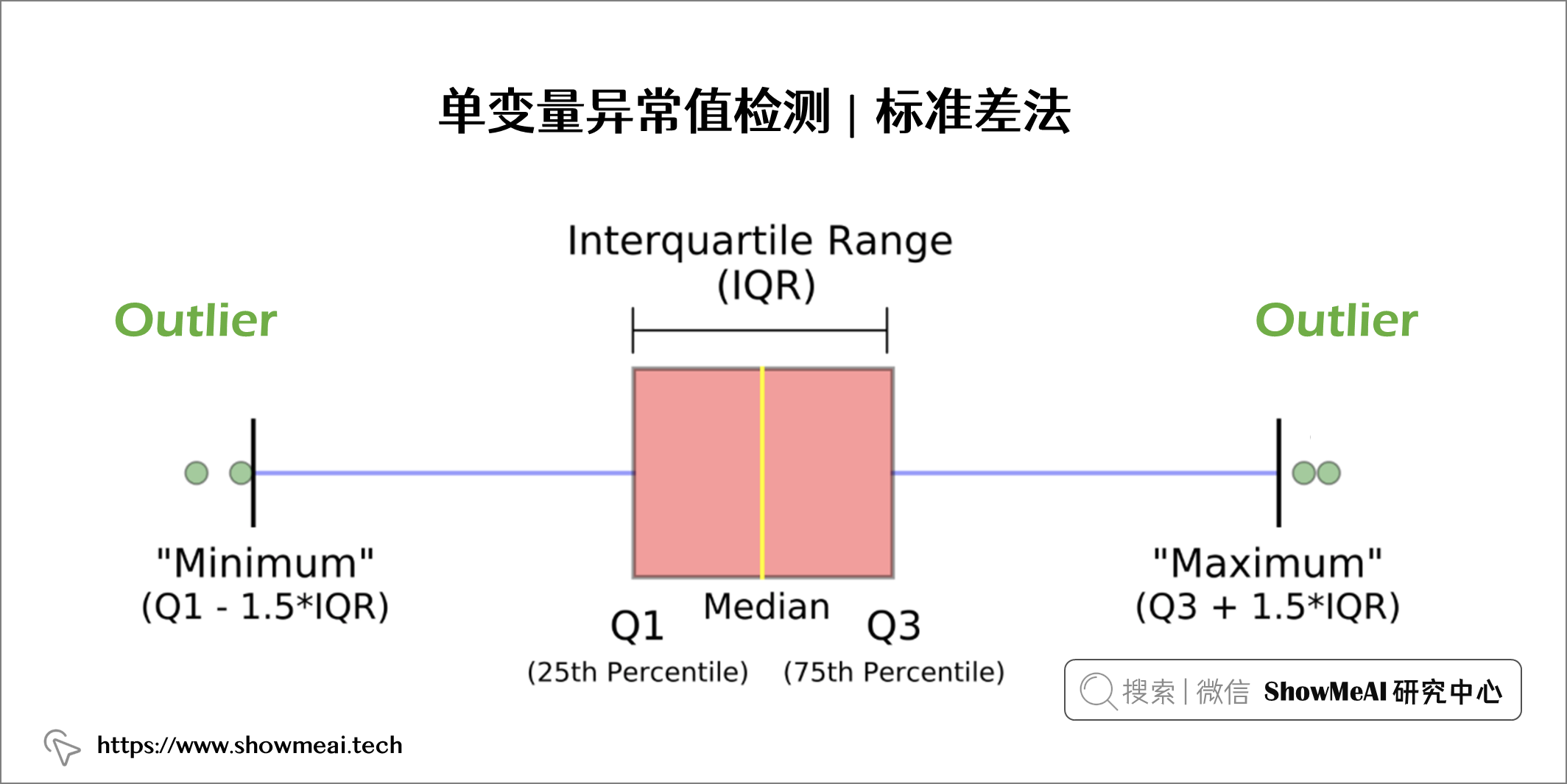```python

# Find Q1, Q3, IQR and cut off value

q25, q75 = np.quantile(glass["Na"], 0.25), np.quantile(glass["Na"], 0.75) iqr = q75 - q25 cutoff = 1.5 * iqr

# Define lower and upper boundaries

lower, upper = q25 - cutoff, q75 + cutoff

# Define new dataset by masking upper and lower boundaries

new_glass = glass[(glass["Na"] > lower) & (glass["Na"] < upper)] Shape of original dataset: (213, 9) Shape of dataset after removing outliers in Na column: (206, 9) ```

# 💡 多變數異常值檢測

## 💦 孤立森林演算法-Isolation Forest

📘孤立森林 是一種基於隨機森林的無監督機器學習演算法。我們都知道，隨機森林是一種整合學習模型，它使用基模型（比如 100 個決策樹）組合和整合完成最後的預估。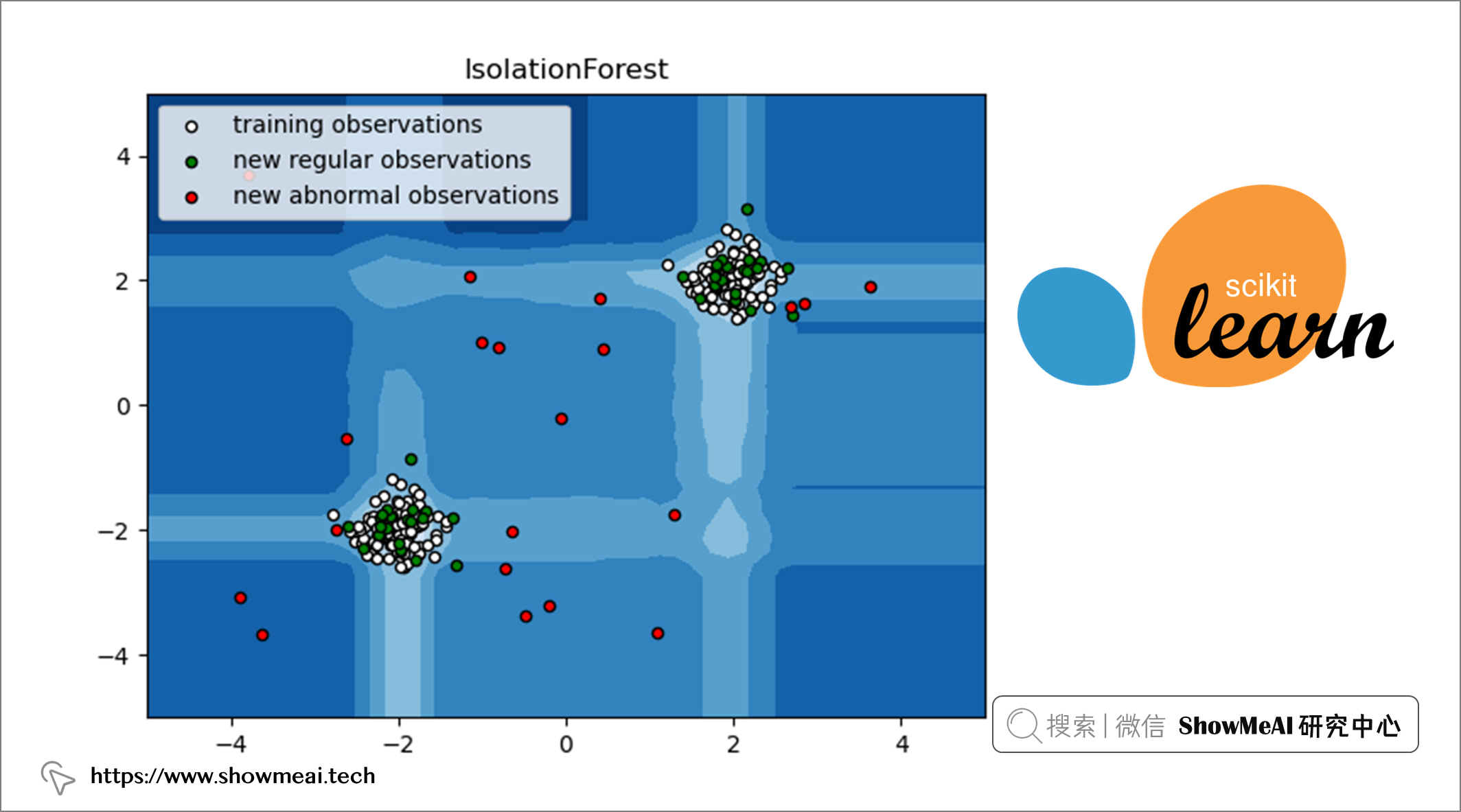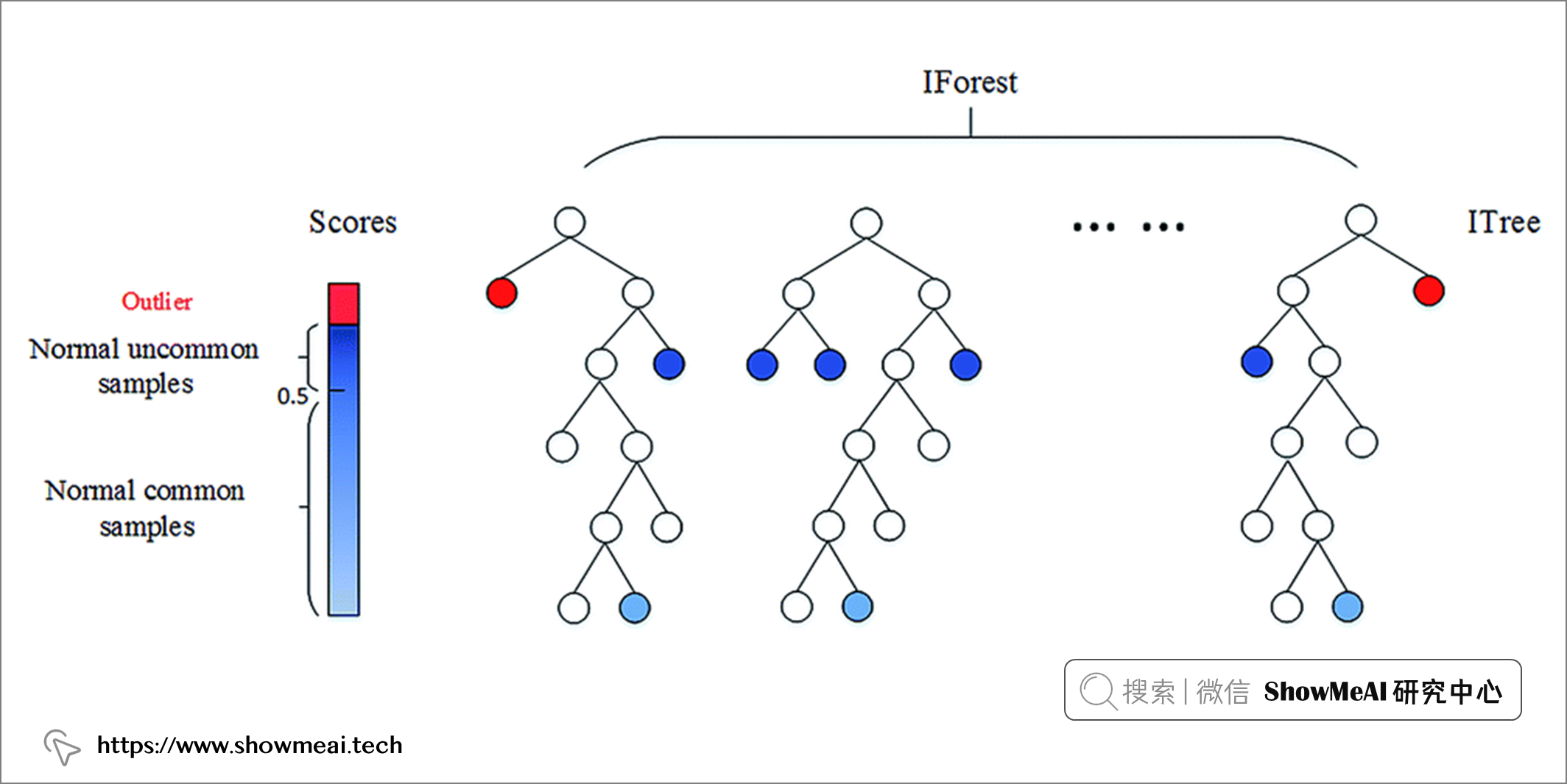```python from sklearn.ensemble import IsolationForest IsolationForest(n_estimators=100, max_samples='auto', contamination='auto', max_features=1.0, bootstrap=False, n_jobs=None, random_state=None, verbose=0, warm_start=False)```

Isolation Forest 演算法有幾個超引數：

• `n_estimators`：表示要整合的基模型的數量。
• `max_samples`：表示用於訓練模型的樣本數。
• `contamination`：用於定義資料中異常值的比例。
• `max_features`：表示取樣處的用於訓練的特徵數。

```python from sklearn.ensemble import IsolationForest

# Initiate isolation forest

isolation = IsolationForest(n_estimators=100, contamination='auto', max_features=glass.shape)

# Fit and predict

isolation.fit(glass) outliers_predicted = isolation.predict(glass)

# Address outliers in a new column

glass['outlier'] = outliers_predicted ```

```1 195 -1 19 Name: outlier, dtype: int64```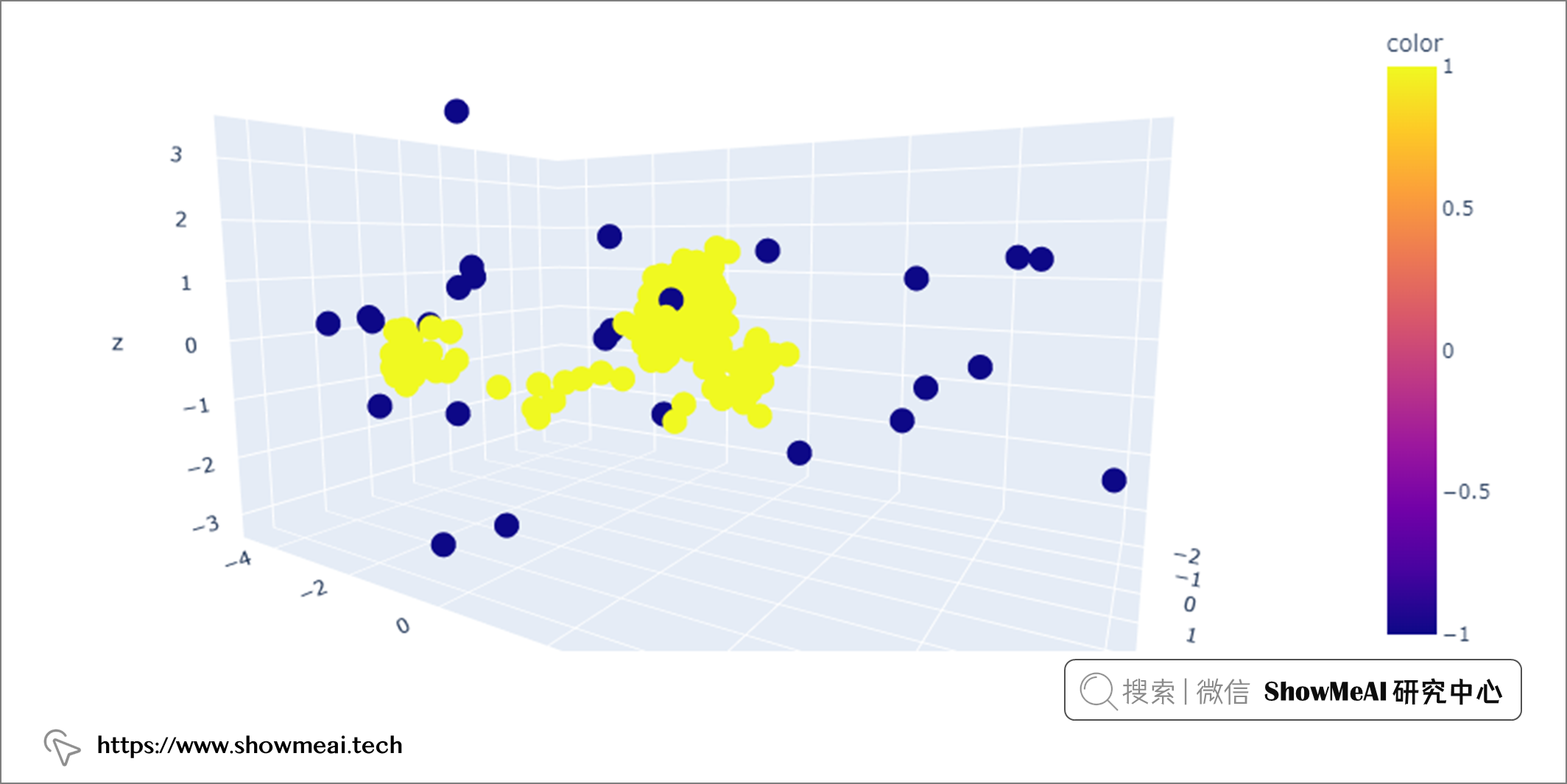## 💦 基於空間密度的聚類演算法-DBSCAN

📘DBSCAN 是一種流行的聚類演算法，通常用作 K-means 的替代方法。它是基於分佈密度的，專注於許多資料點所在的高密度區域。它通過測量資料之間的特徵空間距離（即歐氏距離）來識別哪些樣本可以聚類在一起。DBSCAN 作為聚類演算法最大的優勢之一就是我們不需要預先定義聚類的數量。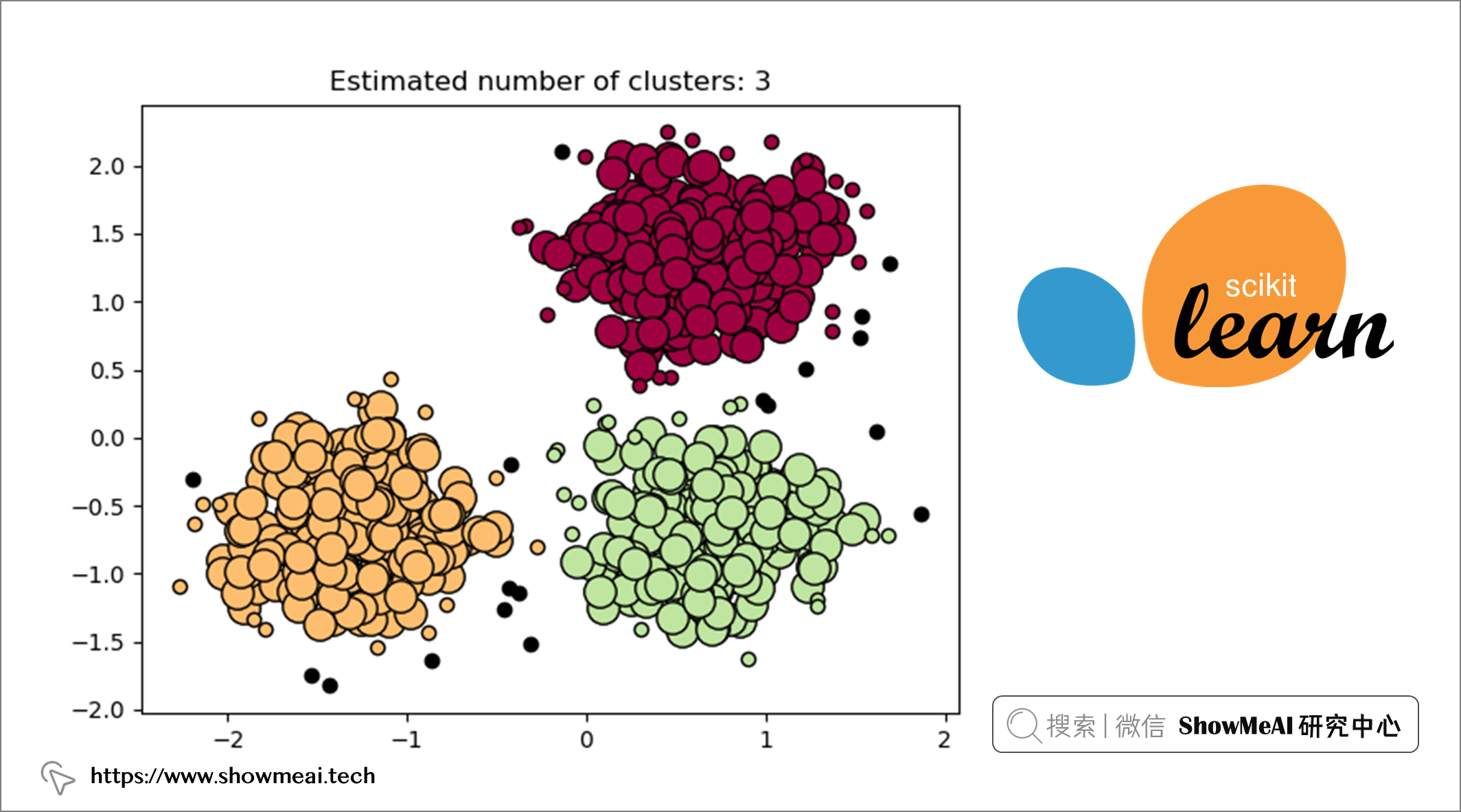```python from sklearn.cluster import DBSCAN DBSCAN(eps=0.5, min_samples=5, metric='euclidean', metric_params=None, algorithm='auto', leaf_size=30, p=None, n_jobs=None)```

DBSCAN 有幾個超引數：

• `eps`（epsilon）：考慮在同一個 cluster 中的兩個資料點之間的最大距離。
• `min_samples`：核心點的接近資料點的數量。
• `metric`：用於計算不同點之間的距離度量方法。

```python import numpy as np from sklearn.cluster import DBSCAN from sklearn.preprocessing import MinMaxScaler

# Initiate DBSCAN

dbscan = DBSCAN(eps=0.4, min_samples=10)

# Transform data

glass_x = np.array(glass).astype('float')

# Initiate scaler and scale data

scaler = MinMaxScaler() glass_scaled = scaler.fit_transform(glass_x)

# Fit DBSCAN on scaled data

dbscan.fit(glass_scaled)

# Address outliers in a new column

glass['outlier'] = dbscan.labels_ ```

```python import math

# Function to calculate k distance

def calculate_k_distance(X,k):

``````k_distance = []
for i in range(len(X)):
euclidean_dist = []
for j in range(len(X)):
euclidean_dist.append(
math.sqrt(
((X[i,0] - X[j,0]) ** 2) +
((X[i,1] - X[j,1]) ** 2)))

euclidean_dist.sort()
k_distance.append(euclidean_dist[k])

return k_distance
``````

# Calculate and plot epsilon distance

eps_distance = calculate_k_distance(glass_scaled, 10) px.histogram(eps_distance, labels={'value':'Epsilon distance'}) ```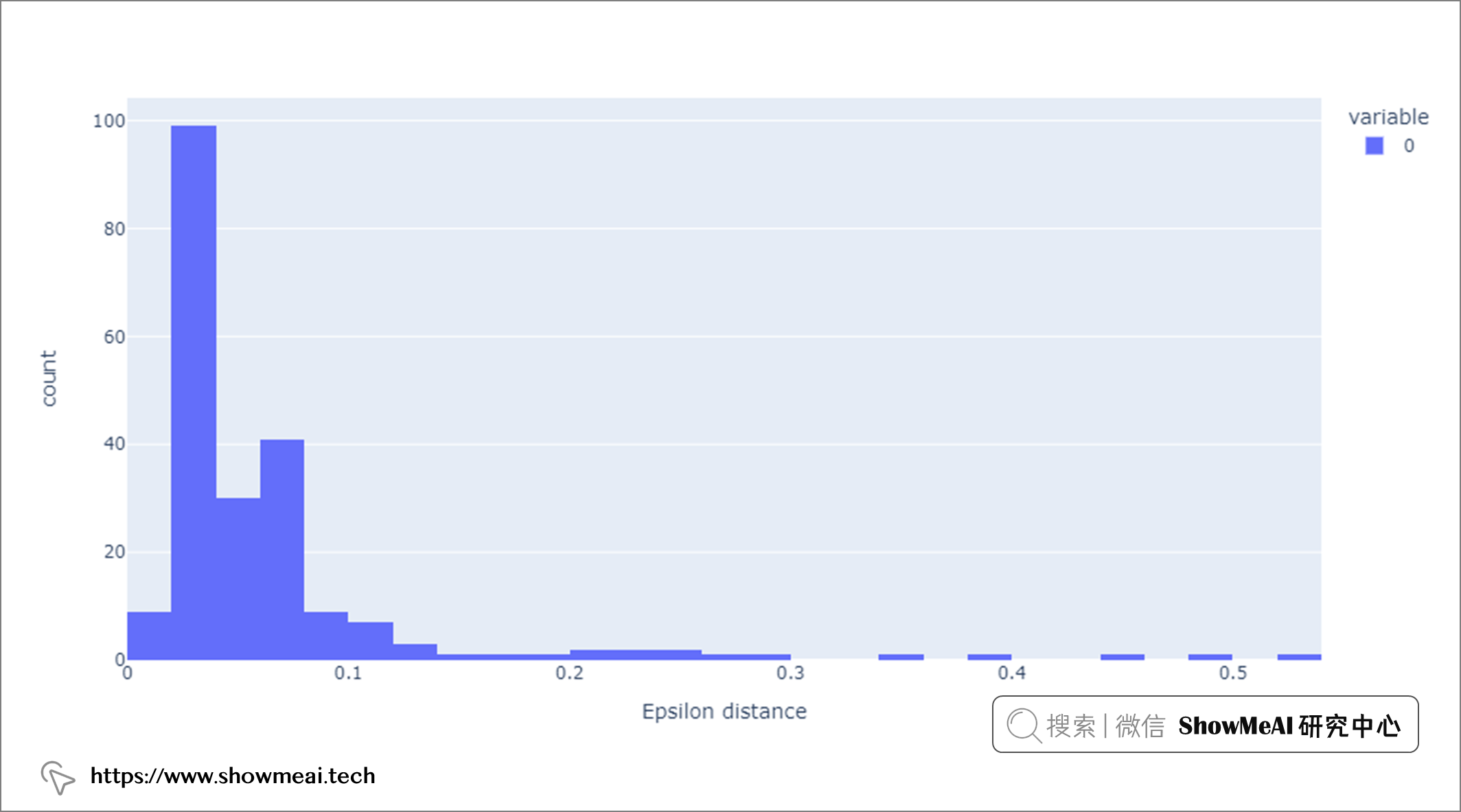```0 192 -1 22 Name: outlier, dtype: int64```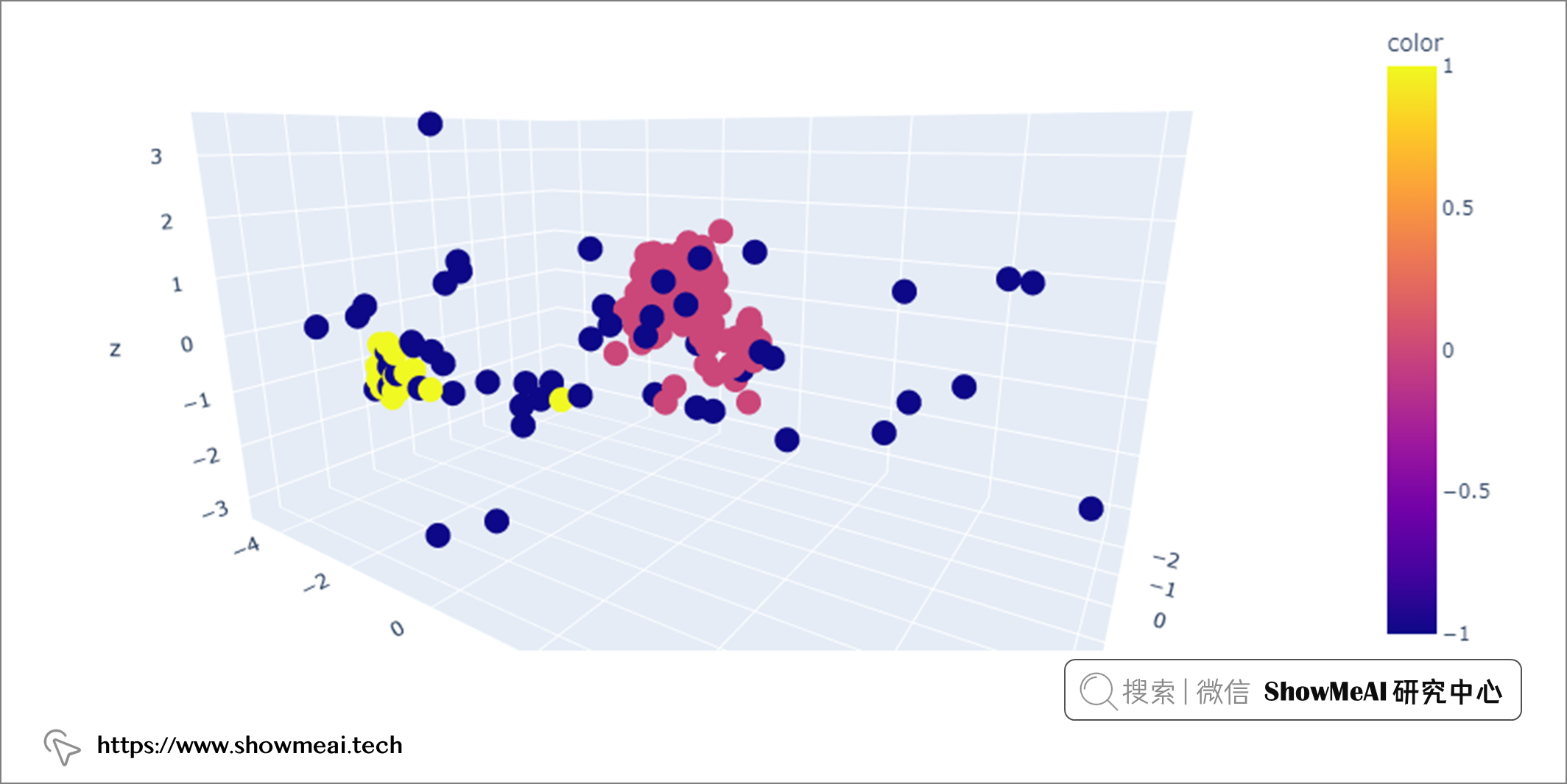## 💦 區域性異常因子演算法-LOF

📘LOF 是一種流行的無監督異常檢測演算法，它計算資料點相對於其鄰居的區域性密度偏差。計算完成後，密度較低的點被視為異常值。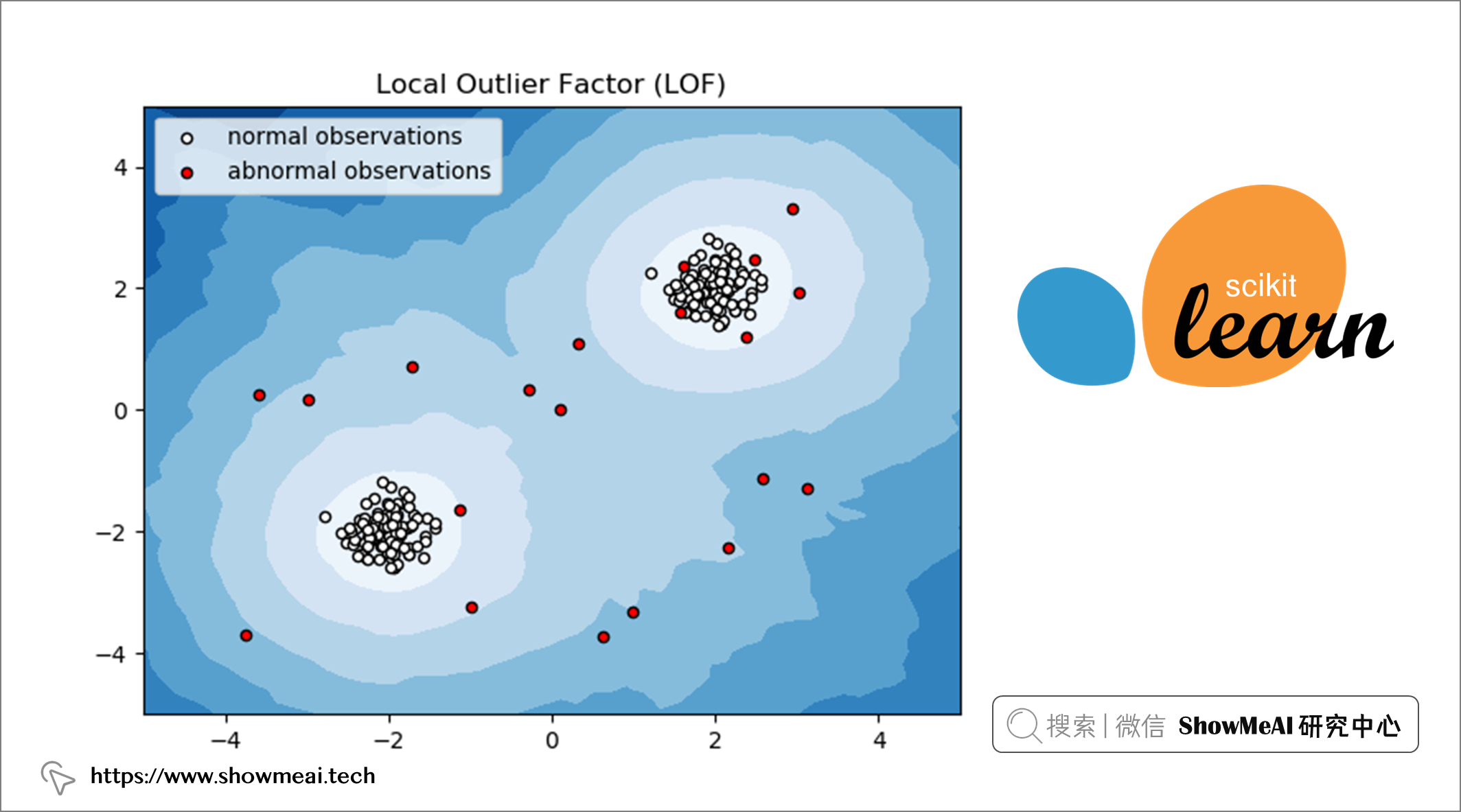```python from sklearn.neighbors import LocalOutlierFactor LocalOutlierFactor(n_neighbors=20, algorithm='auto', leaf_size=30, metric='minkowski', p=2, metric_params=None, contamination='auto', novelty=False, n_jobs=None)```

LOF 有幾個超引數：

• `n_neighbors`：用於選擇預設等於 20 的鄰居數量。
• `contamination`：用於定義離群值比例。

```python from sklearn.neighbors import LocalOutlierFactor

# Initiate LOF

lof = LocalOutlierFactor(n_neighbors=20, contamination='auto')

# Transform data

glass_x = np.array(glass).astype('float')

# Initiate scaler and scale data

scaler = MinMaxScaler() glass_scaled = scaler.fit_transform(glass_x)

# Fit and predict on scaled data

clf = LocalOutlierFactor() outliers_predicted = clf.fit_predict(glass)

# Address outliers in a new column

glass['outlier'] = outliers_predicted ```

LOF建模完成後，通過呼叫`glass['outlier'].value_counts()`我們可以看到有 34 條記錄被標記為`-1`（異常值），其餘 180 條記錄被標記為`1`（正常值）。

```1 180 -1 34 Name: outlier, dtype: int64```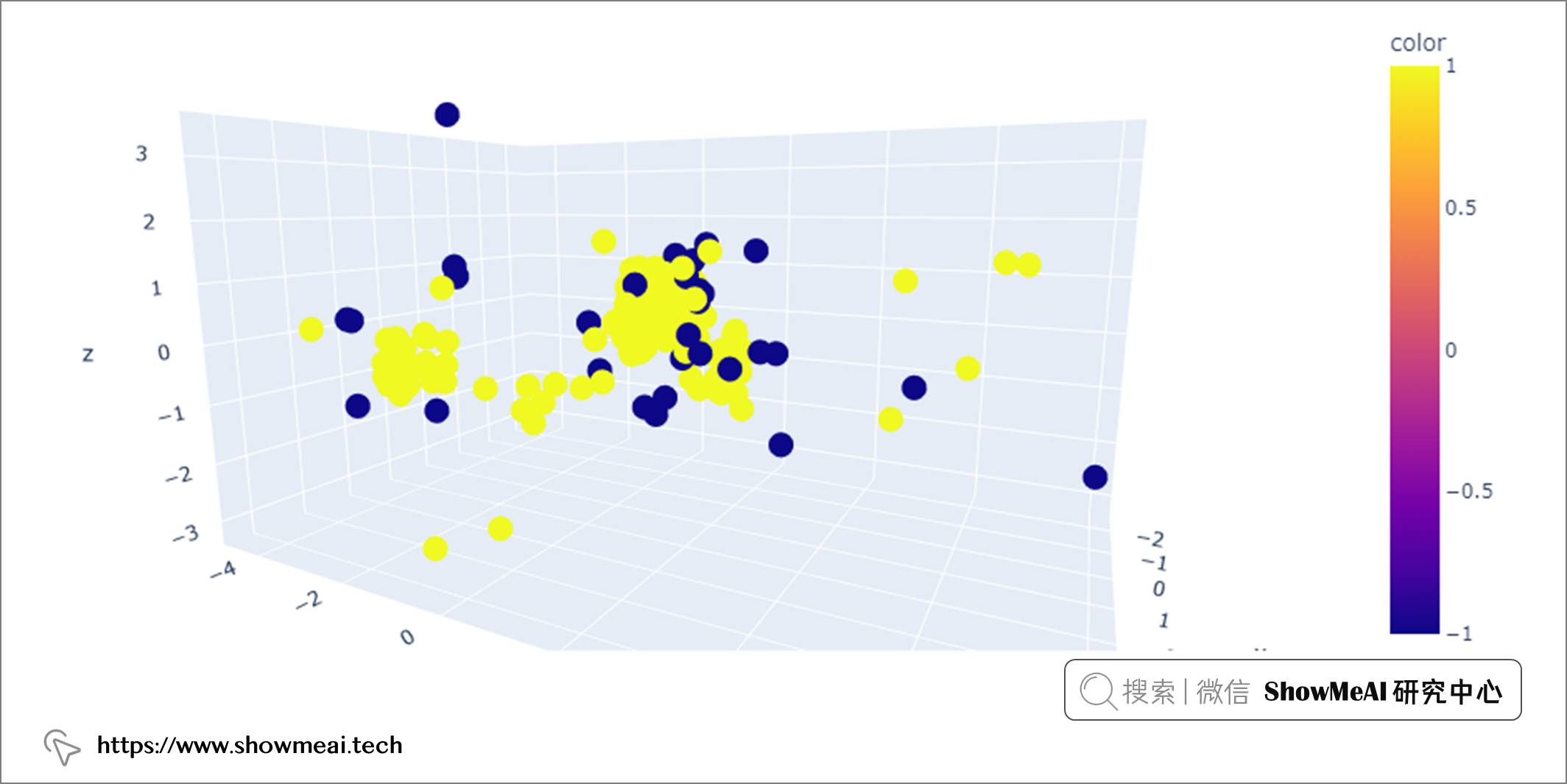# 推薦閱讀